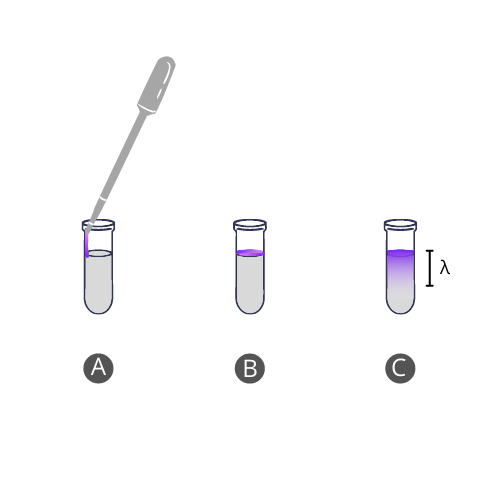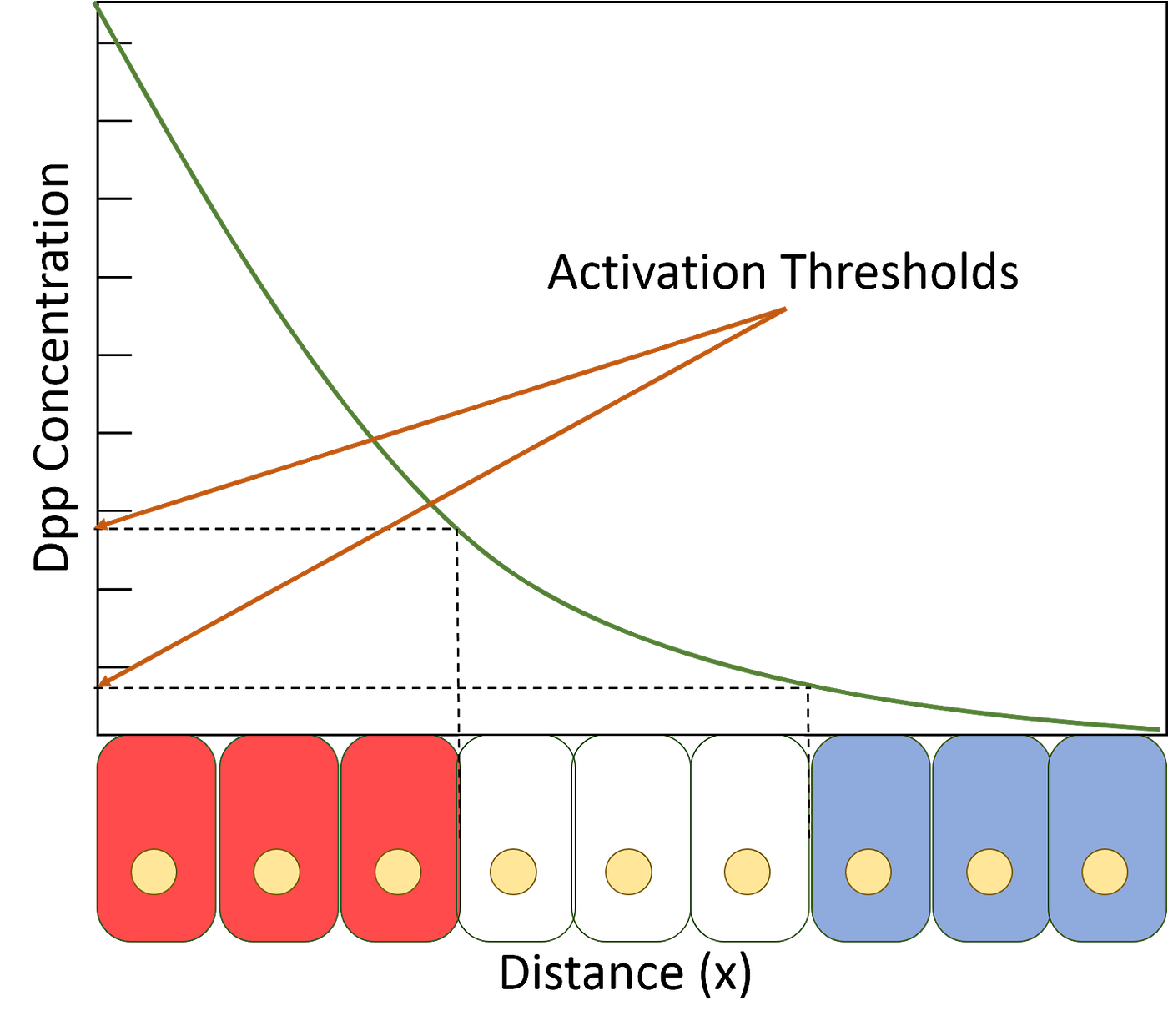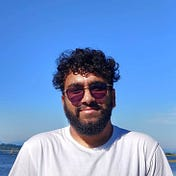# Engineering an Intracellular Differentiation Circuit for Pluripotent StemCells Based on Spatial Location

## Acknowledgement

This paper was an assignment of my introductory biomedical engineering course that I am particularly proud of. This was co-authored by myself and two other students in the class.

# Abstract

To investigate and engineer an intracellular differentiation circuit for pluripotent stem cells based on its spatial and temporal location. With manipulation of cellular distancing and upon activation of bone morphogenetic protein 4 (BMP4), stem cells differentiate into two regions: non-neural brachyury (BRA) and neural sex determining region Y-box 2 (SOX2) positive cells.

# Introduction

The overall goal of this project is to build a one dimensional cell culture that differentiates into certain cell types depending on its spatial and temporal location. A one dimensional cell culture system is simulated by the usage of a linear microtubule with a very small interior diameter in order to expose each subsequent cell in the system to the diffusion of BMP4, sequentially. Furthermore, in order to introduce differential signals so that the cells can proliferate into the desired cell type. Cell behaviour is controlled by a concentration gradient of BMP4 for controlling cellular response.

The BMP4 ligand (L) was chosen since it can be bound to the BMPR2 receptor (R) on the cell membrane of the cells being cultured to form the receptor-ligand complex (C). The BMP4 ligand diffuses throughout the colony of cells by forming a long range concentration profile. Moreover, mathematical models that can predict the dynamic parameters of the ligand can be made by critically analyzing such profiles. One such model that must be solved is:

A pertinent observation that is critical to study is the linearly proportional relationship between BMPR2 (R) on the cell membrane and the concentration of BMP4 (L) outside the cell. Two quantifiable measurements determined prior to the investigation of this study are: diffusion coefficient constant of 1.2 m2/sand its effective degradation rate as kR = 5.4*10–5s-1.

The exact objective of this study was to design a system wherein cells differentiate into BRA positive cells within a certain distance from the BMP4 edge that activates the protein and cells greater than the decay length differentiate into SOX2 positive cells. The use of differential equations can help model and predict the exact behaviour of cells in such an environment.

Since it is known that for large cell population (N) there is a high concentration of the ligand BMP4, amd since BMP4 is proportional to BMPR2 by some proportionality constant. We need to create a signal (S) that increases with N, and a growth rate ; thus one needs to solve:

## Methodology used to solve the problem.

The first step in solving this problem is to develop a standard method of creating a one dimensional (1D) cell culture. Specifically for this experiment, a linear microtube with a very small interior diameter is utilized in order to expose each subsequent cell in the system to the diffusion of BMP4 sequentially. In regards to the performance of an accurate simulation in one dimension, BMP4 is administered to the system by pipetting a very small amount of a medium in which BMP4 has much lower solubility in comparison to the original solution and dispensing it on the walls of the microtube, thus allowing for a very thin layer of protein on top of the cell culture solution. This creates a concentration gradient throughout the entire microtube through a diffusion flux.Figure 1. Apparatus diagram for the setup of administering BMP4 to the cell culture.

## Design of the tools and process to solve the problem.

In order to calculate the decay length , one must consider Fick’s second law. The diffusion equation shows the macroscopic dynamics of the concentration of ligands, and can be derived by applying mass conservation to Fick’s first law.

At long times the concentration profile reaches an out-of-equilibrium steady state and we can set the rate of ligands over time to zero. Furthermore, the decay of ligands is a dying exponential function controlled by the decay gradient. Using both of these pieces of information, one can solve for the decay length, , by knowing the diffusion constant, D, and the rate of degradation, k.

The next step in designing this intracellular differentiation circuit is to solve eq. (1), eq. (2), and eq. (3). These solutions will provide valuable information concerning concentration of the ligands and receptors. Additionally, since it is known that BMP4, the ligand, and BMPR2, the receptor, are linearly proportional that can be described by eqn. (4). For long periods of time the concentration reaches a steady state, we can then solve for the respective concentration. We set eq. (1), eq. (2), and eq. (3) to 0 and solve for R, L and C respectively.

One can further examine the differentiation circuit by calculating the concentration of the ligand-receptor complex in steady state from the source when the position is exactly λ μm away. It is at this boundary that the cells should differentiate into SOX2+ cells instead of BRA+ cells. This can be accomplished by using eq. 11 and eq. 8 and eq. 4. By setting x = λ and solving L(λ)one gets L₀/e, using this one can also solve for R, αL₀/e. We plug these two results to get the following equation:

One specific network motif that can model the current cell circuit is a simple model where: BMP4 activates BRA+ cells however activates SOX2+ instead if a certain concentration threshold is not met. This simple model describes the pattern for different concentrations of the ligand-receptor complex such that in the region of the microtube with concentrations of BMP4 in excess of approximately 7.358e-10ng/𝜇m, cells differentiate into BRA positive cells, while in the region of the microtube with concentrations of BMP4 below that of 7.358e-10ng/𝜇m, cells differentiate into SOX2 positive cells. For example, in the case of initial ligand concentration L₀=2ng/𝜇m, this corresponded to a displacement of 150𝜇m from which BMP4 was administered.

# Results

One can determine solutions to the steady state approximation of the receptor and ligand equations considering eq. 9 and eq. 10. Moreover, given the initial concentration of L, the concentration of R can be found through eq. 4. This computation returns an expression of:

# Discussion

One certain aspect that one must consider in order to create an adaptable and scalable is how would one modify the circuit such that the region of BRA+ is changed. One possible way to accomplish this task is by choosing a more suitable growth factor. This way, the diffusion constant changes and so does the degradation rate. This change will affect the overall decay length, cells beyond the decay length will differentiate into SOX2+. Refer to Figure 2 for a graphical representation of this phenomenon.Figure 2. Graph of concentration of BMP4 as a function of distance (red cells are BRA+, blue cells are SOX2+, activation threshold is λ, white cells are modifiable region for BRA+)

Thus, the region of BRA+ cells is directly controlled by the decay length, as the entire differentiation circuit is contingent on the spatial location of the cell itself and its relative proximity to the source of BMP4. Therefore, it makes sense that the diffusion constant and degradation rate dictate the overall region of BRA+ cells.

A second method involves the consideration of eq. 8. Since the concentration of the ligand at x 𝜇m is proportional to L₀, it follows that one could increase the initial concentration of the BMP4 solution administered into the cell culture, thereby increasing the displacement x at which L(x) attains the value of 7.385e-10ng/𝜇m.

Another critical aspect of creating this cell differentiation circuit is to be able to control the sensitivity of cell proliferation. This was modelled by eq. 5, eq. 6, and eq 7. The cell population from eq5. has a solution of the type:

The solution is described by N0, initial cell population, and , growth rate. Mimicking the aforementioned network motif, the solution is simply a positive function that is controlled by λ. Lastly, in order to describe the growth rate, one could utilize Michaelis-Menten and Lineweaver-Burk1 equations to describe the growth rate as a cycle that depends on the growth medium, G (I). Additionally, one could also create a function where growth rate depends on S and a parameter that modulates how much S one needs to have =0.5(II).

The replication of a cell is undoubtedly heavily influenced upon its differentiation patterns, and control of such parameters can, and already has, had a significant impact on the field of therapeutics. One particular example shows the cell differentiation of mesenchymal stem cells being able to develop into several different types of tissue depending on how their differentiation is directed. This one application of cellular differentiation holds a wide scope of usage, such as the mitigation of osteoporosis.

The applications are not limited to the manipulation of stem cells in the body, but differentiation patterns apply to all living organisms. One obvious example is bacterial organisms. Increased understanding of how the replication of bacterial matter operates, the better one is able to prevent such replication. This is the concept that many antibodies function off of, binding to receptor sites to prevent further replication. Another method of treatment with common bacteria such as E. coli is the inhibiting of DNA A, a protein responsible for the activation of DNA replication. With an adequate understanding of how replications occur, various medicines or even viral cures can be made to oppose such replication.

# Conclusion

It was found that a decay length of 149m allowed for the differentiation circuit to be modeled by partial differential equations. Additionally, when L₀=2ng/𝜇m, α=5.1*10–3 and kR=10–3; then receptor ligand equations could be modelled as described in this study.

# References

 Chen, C.-H.; Chen, S. C. Cell Growth Factor Activity. Experimental Cell Research 1981, 136 (1), 43–51.

--

--## Muhammad Umar Ali

I am an undergraduate student studying biomedical engineering at the University of British Columbia. Check out umarali.ca and my GitHub for more!